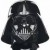# attribute response DOE using logistic regression?

Six Sigma – iSixSigma Forums Old Forums General attribute response DOE using logistic regression?

Viewing 3 posts - 1 through 3 (of 3 total)
• Author
Posts
• #51503

Hello
Just want some advice from those pretty well-versed in stats.   Without going into too much detail, I have a ‘traditional’ two-level factorial DOE with an attribute response.  That is, “defective” or “non-defective”.  No way to get around this one unfortunately.  Anyway, some of the traditional ways for analysis include analyzing the percentages almost like this was variable data, with an improved approach being to take a transform of the percentages (ex. inverse sine) or something to that effect to stabilize variance.  Of course, there’s nothing better than graphical analysis, so this is done as well…  Another approach that I have been considering is logistic regression.  I do get essentially the same results with both approaches.
However, if anybody has some experience with this or can tell me if there’s any significant flaws in this logic, please let me know.  The factors are both attribute and variable as well, which is generally fine for regression.  Thank you.  JP

0
#178640Ken Feldman
Participant

JP,I have tried to post a response twice already but it is not getting through. I have some links that could be helpful. I found them by googling “attribute response DOE”. Try that and you will likely find the same links I did and they made help you a bit.

0
#178649Robert Butler
Participant

When you say you “get essentially the same results with both approaches.”  I’m assuming the two approaches are linear regression vs. logistic regression and the same results are the significance or lack thereof of the variables of interest.
If this is what you mean then while the same terms may have appeared in the final stepwise model for the linear regression and the logistic regression the way you use what you have from the two approaches is different.  In the standard regression the coefficients are associated with terms in a predictive model where the model is used to predict an outcome (percent defective/non-defective) based on the way the inputs are changed.  The results of a logistic regression are point estimates of odds ratios.  These ratios are not coefficients in the sense of a linear regression.
Your comments concerning data transformation and linear regression are in accordance with recommended practice (pp. 123-145 Box Hunter and Hunter Statistics For Experimenters – 2nd Edition).  Depending on the range of the Y the transformation may or may not be of any benefit.  If your object is to develop a regression equation I’d recommned the usual regression analysis (residual checks etc.) to check the need for transformation and model adequacy and I’d avoid logistic regression.

0
Viewing 3 posts - 1 through 3 (of 3 total)

The forum ‘General’ is closed to new topics and replies.